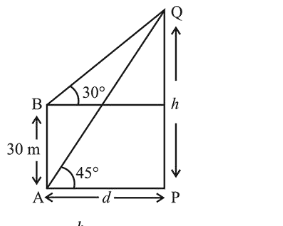# The angle of elevation of the top of a verticalQuestion:

The angle of elevation of the top of a vertical tower standing on a horizontal plane is observed to be $45^{\circ}$ from a point $\mathrm{A}$ on the plane. Let $\mathrm{B}$ be the point $30 \mathrm{~m}$ vertically above the point $\mathrm{A}$. If the angle of elevation of the top of the tower from $\mathrm{B}$ be $30^{\circ}$, then the distance (in $\mathrm{m}$ ) of the foot of the tower from the point $\mathrm{A}$ is:

1. (1) $15(3+\sqrt{3)}$

2. (2) $15(5-\sqrt{3)}$

3. (3) $15(3-\sqrt{3)}$

4. (4) $15(1+\sqrt{3})$

Correct Option: 1

Solution:

Let the height of the tower be $h$ and distance of the foot of the tower from the point $A$ is $d$.

By the diagram,$\tan 45^{\circ}=\frac{h}{d}=1$

$h=d$ ...(i)

$\tan 30^{\circ}=\frac{h-30}{d}$

$\sqrt{3}(h-30)=d$....(ii)

Put the value of $h$ from (i) to (ii),

$\sqrt{3} d=d+30 \sqrt{3}$

$d=\frac{30 \sqrt{3}}{\sqrt{3} 1}=15 \sqrt{3}(\sqrt{3}+1)=15(3+\sqrt{3})$